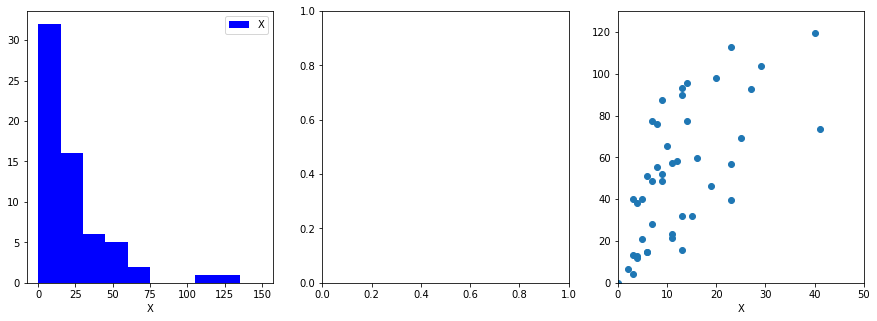# Data Visualization

Back To Index

02_DataVisualization

## Data Visualization¶

A modern data scientist relies on several visualization techniques to sniff out trends, outliers and patterns in data. A typical visualization exercise uses constructs like graph plots, bar/pie charts, scatter plots and historgrams. Here we will use histograms to look at the distribution of variables in our dataset, and a scatter plot to look at the correlation amongst them.

### Dataset¶

We will use Swedish isurance dataset taken from following source (Reference: Swedish Committee on Analysis of Risk Premium in Motor Insurance): https://college.cengage.com/mathematics/brase/understandable_statistics/7e/students/datasets/slr/frames/frame.html

The data has two columns:

X = number of claims

Y = total payment for all the claims in thousands of Swedish Kronor for geographical zones in Sweden

In :
###################################################
#  Filename : data_visualization                  #
#  Purpose : To demonstrate use of matplotlib     #
#   for creating simpel visuals                   #
#            1. Histograms                        #
#            2. Scatter Plots                     #
#  Author : Niel S.                               #
#  The English Tea Company LLC                    #
###################################################
from matplotlib import pyplot as plt

#The Dataset.

#These are the values of the independent variable.
X = [108,19,13,124,40,57,23,14,45,10,5,48,11,23,7,2,24,6, \
3,23,6,9,9,3,29,7,4,20,7,4,0,25,6,5,22,11,61,12,4,16,13,60,\
41,37,55,41,11,27,8,3,17,13,13,15,8,29,30,24,9,31,14,53,26]

#These are the values of the dependent variable
Y = [392.5,46.2,15.7,422.2,119.4,170.9,56.9,77.5,214,65.3, \
20.9,248.1,23.5,39.6,48.8,6.6,134.9,50.9,4.4,113,14.8, \
48.7,52.1,13.2,103.9,77.5,11.8,98.1,27.9,38.1,0,69.2,14.6, \
40.3,161.5,57.2,217.6,58.1,12.6,59.6,89.9,202.4,181.3,152.8, \
162.8,73.4,21.3,92.6,76.1,39.9,142.1,93,31.9,32.1,55.6,133.3, \
194.5,137.9,87.4,209.8,95.5,244.6,187.5]

def main():
#Visualizing the data.
visualize_input_data(X,Y)

def visualize_input_data(X,Y):
'''
Input:
X : a column of x values.
Y : a column of y values.
'''

#Create a figure object with 3 axes.
fig0,(ax0,ax1,ax2) = plt.subplots(1,3,figsize=(15,5))

#How many bins we need for histograming?
max_range = 150
min_range = 0
bin_width = 15
####Number of bins
nbins =int((max_range-min_range)/bin_width)
####Bins
bins = [0+i*bin_width for i in range(0,nbins+1)]

#Create the histogram a.k.a frequency chart of X
n0,b0,p0 = ax0.hist(X,bins=bins,histtype='stepfilled',label='X',color='blue')

#Create a histogram of Y (same binning as in X).
'''
Exercise: Code for Y histogram goes here.
'''

#Some Cosmetic settings for ax0
ax0.set_xlabel('X')
'''
Exercise: Add code to set Y label to 'Frequency'
'''
ax0.legend()

#Some Cosmentics settings for ax1
'''
Exercise: Add Code to do exactly same
settings for ax1 as ax0
'''
##Do a scatter plot for the variables.
ax2.scatter (X,Y,label='Weekly Returns')
ax2.set_xlabel('X')
ax2.set_xlim([0,50])
ax2.set_ylim([0,130])
'''
Exercise: Add code to set ylabel as 'Y'
'''
'''
Exercise: Add code to display the legend
'''

if __name__ == '__main__':
main()# Conclusion¶

A look at the histograms show that variable X (number of claims) is concentrated within the range 0-15 for most of the geographical regions, whereas the variable Y ( total payment in thousands ) peaks around the range 25-35. The scatter plot suggests that linear dependence of Y on X with 3.0 of slope.

Back To Index